• 2021-05-05 08:49:37

实验一 典型连续时间信号描述及运算

一、目的和预先知识

1、目的：

(1)通过绘制典型信号的波形，了解这些信号的基本特征

(2)通过绘制信号运算结果的波形，了解这些信号运算对信号所起的作用 (3)通过将信号分解成直流/交流分量，初步了解信号分解过程 2、预先知识：

熟练运用MATLAB 编程语言。

二、典型连续信号波形的绘制 1、基于MATLAB 的信号表示方法

1)向量表示方法

对于连续时间信号)(t f ，可以定义两个行向量f 和t 来表示，其中向量t 是形如21::t p t t =的MATLAB 命令定义的时间范围向量，1t 为信号起始时间，2t 为终止时间，p 为时间间隔。向量f 为连续时间信号)(t f 在向量t 所定义的时间点上的样值。例如对

于连续时间信号t

t t Sa t f )

sin()()(==，可以用如下两个向量表示：

t=-10:1.5:10 f=sin(t)./t

命令执行结果为：

t =

Columns 1 through 7

-10.0000 -8.5000 -7.0000 -5.5000 -4.0000 -2.5000 -1.0000 Columns 8 through 14

0.5000 2.0000 3.5000 5.0000 6.5000 8.0000 9.5000 f =

Columns 1 through 12

-0.0544 0.0939 0.0939 -0.1283 -0.1892 0.2394 0.8415 Columns 13 through 14

0.9589 0.4546 -0.1002 -0.1918 0.0331 0.1237 -0.0079 用上述向量对连续信号进行表示后，就可以用plot 命令绘出该信号的时域波形。plot 命令可将点与点间用直线连接，当点与点间的距离很小时，绘出的图形就成了光滑的曲线。

MATLAB 命令如下： plot(t,f)

title(‘f(t)=Sa(t)’) xlabel(‘t ’)

axis([-10,10,-0.4,1.1])

绘制的信号波形如图1-1所示，当把时间间隔p 取得更小(如02.0=p )时，就可得到)(t Sa 较好近似波形，如图1-2所示。

更多相关内容
• 什么是指数信号？ 解释：我们在中学就知道指数函数，而我在这里所说的指数信号则是 当 a=0 时，信号不随时间变化，成为直流信号 当 a>0 时，信号将随时间增长。 当 a<0 时，信号将随时间衰减。 常数 K...
• 什么是指数信号？

解释：我们在中学就知道指数函数  ，而我在这里所说的指数信号则是

a=0 时，信号不随时间变化，成为直流信号

a>0 时，信号将随时间增长。                              当 a<0 时，信号将随时间衰减。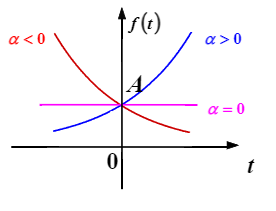常数表示的是指数信号在 t=0 点的初始值。

指数 a 的绝对值大小反映了信号增长或衰减的速度快慢。

指数信号的一个重要特性是：其对时间的微分和积分形式仍为指数形式

 |a|  越大 增长或衰减的速度越快 |a|  越小 增长或衰减的速度越慢
通常情况下，把 |a| 的倒数称为指数信号的时间常数，记作   ，即    ，   越大，指数信号增长或衰减的速度越慢。
• 什么是复指数信号？

解释：复指数信号是相对于指数信号而言的，当指数信号的指数因子为一复数时，称为复指数信号，其表示式为:         ，其中

所以说：• 什么是正弦信号？

解释：首先我们知道在数学中，有余弦函数与正弦函数之分，但是对于余弦信号与正弦信号而言，它们之间的相位仅相差   ，所以我们在信号与系统中，基本上都统称为正弦信号，所以我们在该书中基本上看到余弦信号也被称为正弦信号。

其表达式为   ，其中 A 为振幅， 是角频率， 为初相位。其波形如下图所示：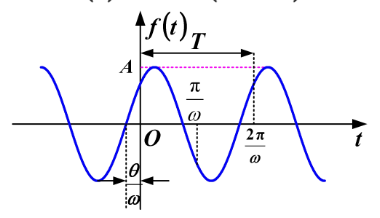除此之外，我们在信号与系统中还遇到一些衰减的正弦信号，如下图所示：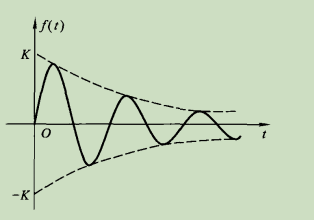其表示式为：

正弦信号与余弦信号可以借助复指数信号来表示。根据欧拉公式可以知道：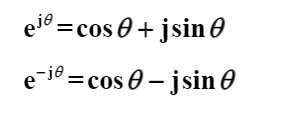所以可以得出：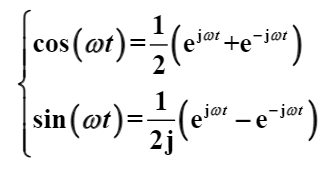• 什么是 Sa(t) 函数（抽样函数）？

解释：Sa(t) 函数即 Sa(t) 信号是指 sint t 之比构成的函数，定义如下：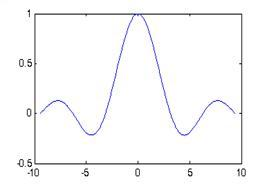函数的性质有：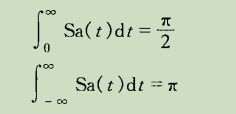Sa(-t) = Sa(t)   为偶函数

与 Sa(t) 函数类似的是 Sinc(t) 函数，其表示式为：

有些书中将两种符号通用，即Sa(t) 也可以用 Sinc(t) 表示。

• 什么是钟形信号（高斯函数） ？

解释：钟形信号（高斯函数）的定义是：

波形如图所示：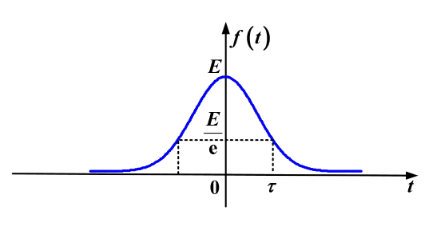展开全文• 连续非周期信号频谱分析及Matlab实现.pdf
• 这一部分我们介绍三个点，如图所示下面分别介绍连续时不变特征信号线性时不变(linear time-invariant)系统特征函数这一系统的信号在微分和积分操作下，函数形式保持不变。对于这样的函数我们称之为该系统的特征函数...

这一部分我们介绍三个点，如图所示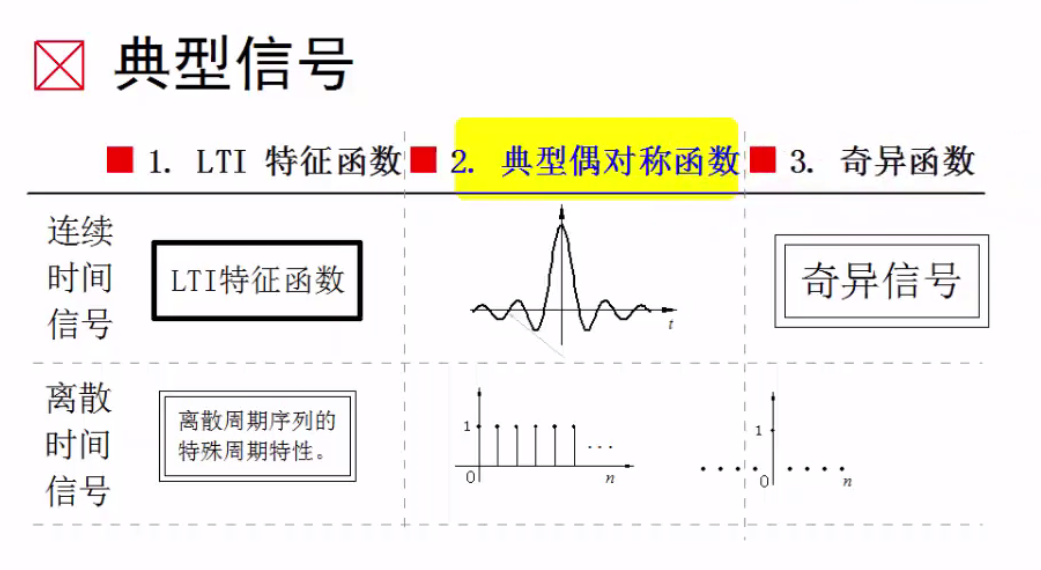下面分别介绍

## 连续时不变特征信号

### 线性时不变(linear time-invariant)系统特征函数

这一系统的信号在微分和积分操作下，函数形式保持不变。对于这样的函数我们称之为该系统的特征函数。我们在理解时可以对比矩阵的特征值和特征向量

Ax=λx

A是某种操作，在这种操作下，特征值和特征向量保持不变。我们还可以想想指数函数，指数函数在微分下指数形式是保持不变的。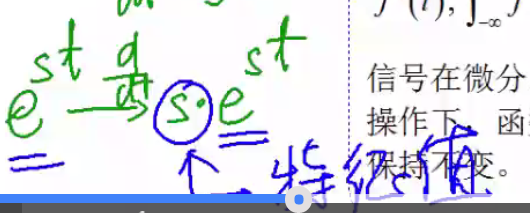一个图概括如下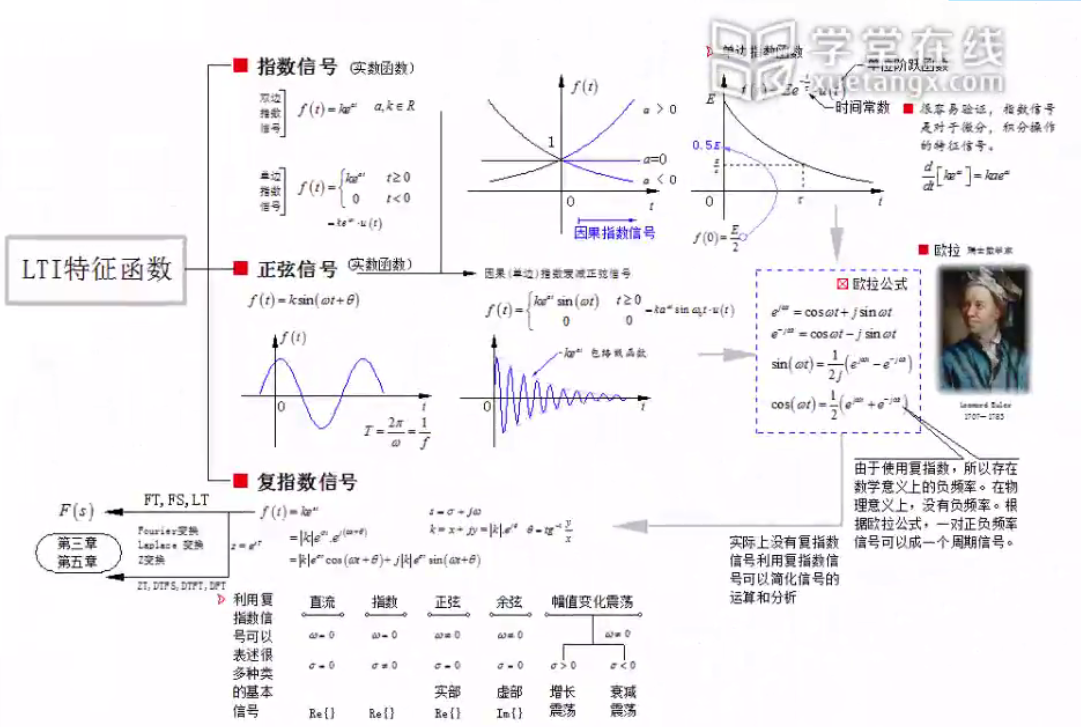## 对称信号

我们在这里介绍的对称信号实际上是一对，他们分别是高斯信号和抽样信号

### 高斯信号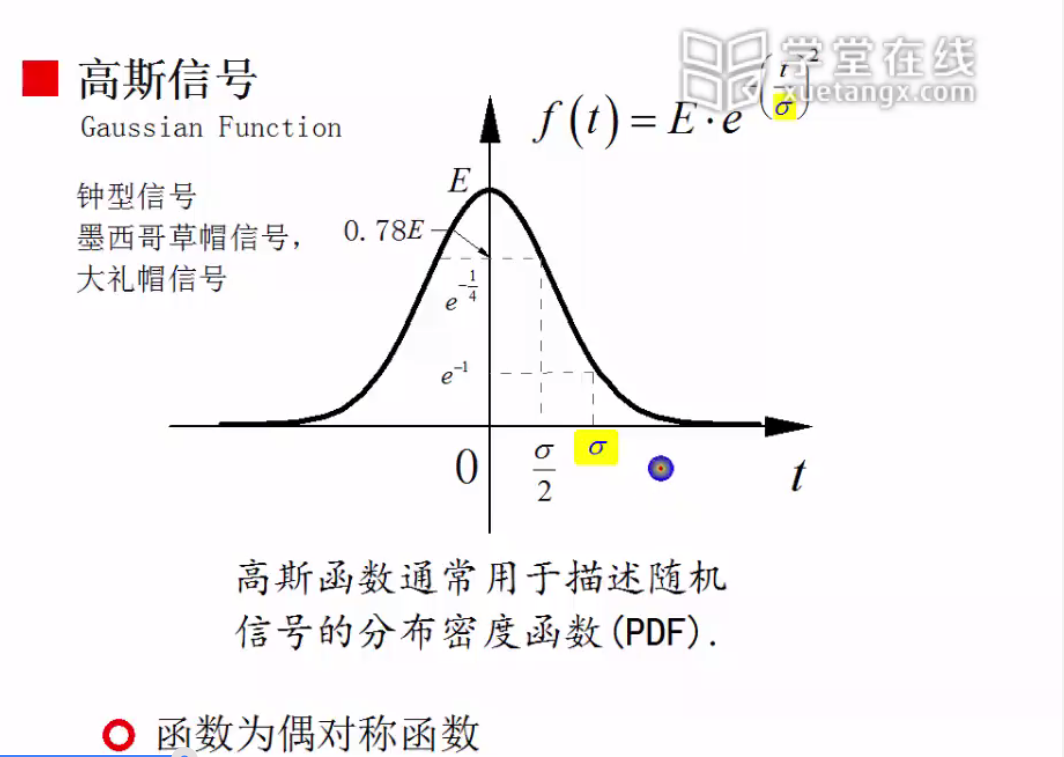注意，高斯信号实际上是一个从负无穷到正无穷一直都存在的信号。但我们知道指数衰减是一种很快的衰减，因此我们可以认为整个信号有效值基本集中在3 σ $\sigma$之间。

函数的性质如图所示：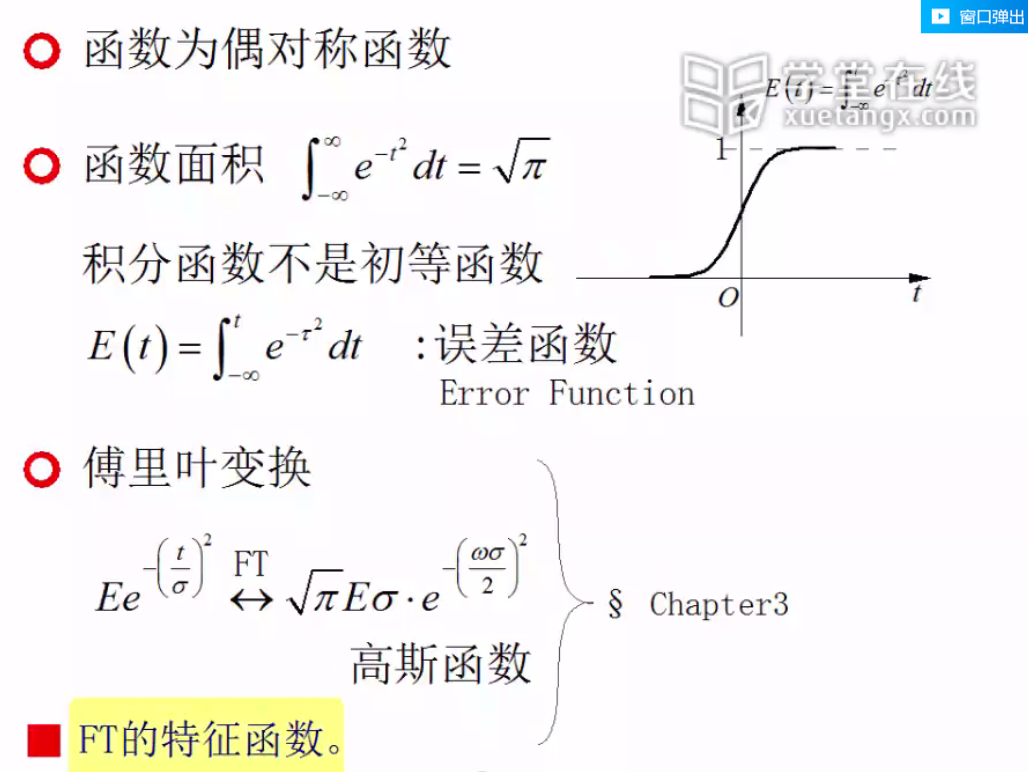### 抽样信号

如图所示为抽样信号的函数形式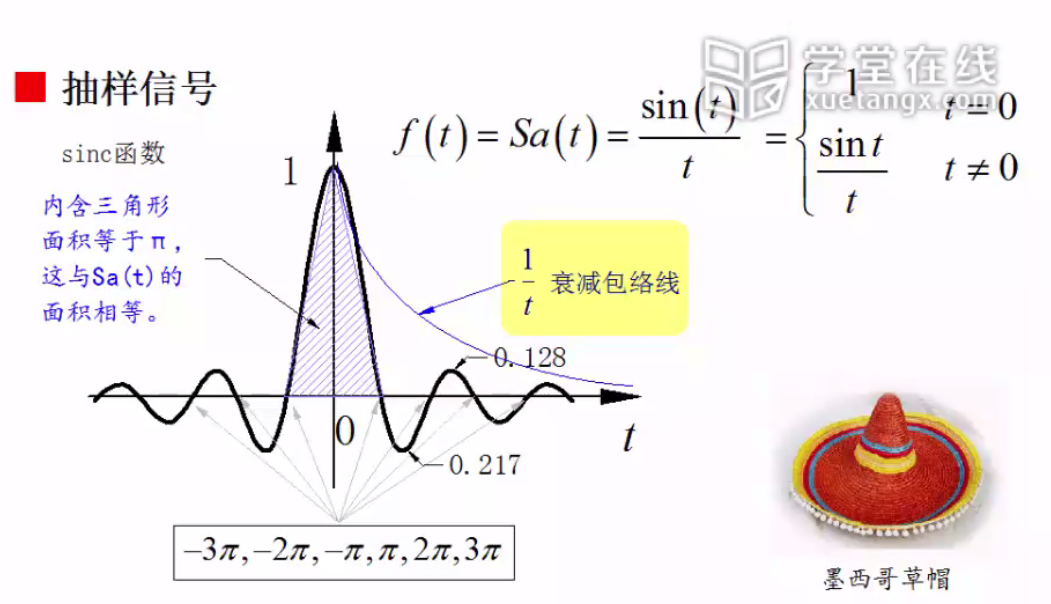函数的性质如下图所示：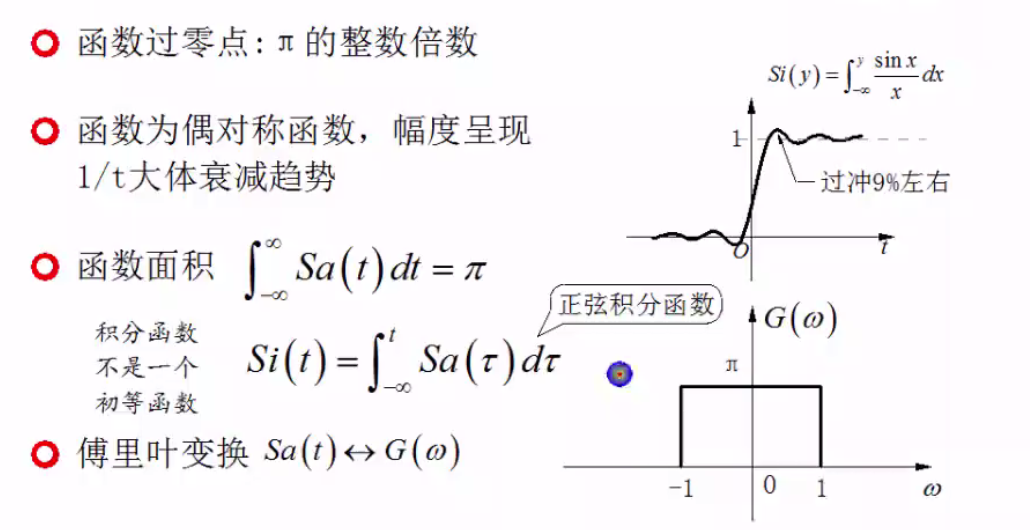# 奇异信号

## 奇异信号介绍

奇异信号是指函数本身或者导数存在不连续点的信号

### 单位斜变信号

如图所示，之所以称斜变信号为奇异信号是因为他的导数是不连续的，负半轴为0正半轴为1.斜变信号有很多应用，最常见的就是老式电视的锯齿形扫描函数。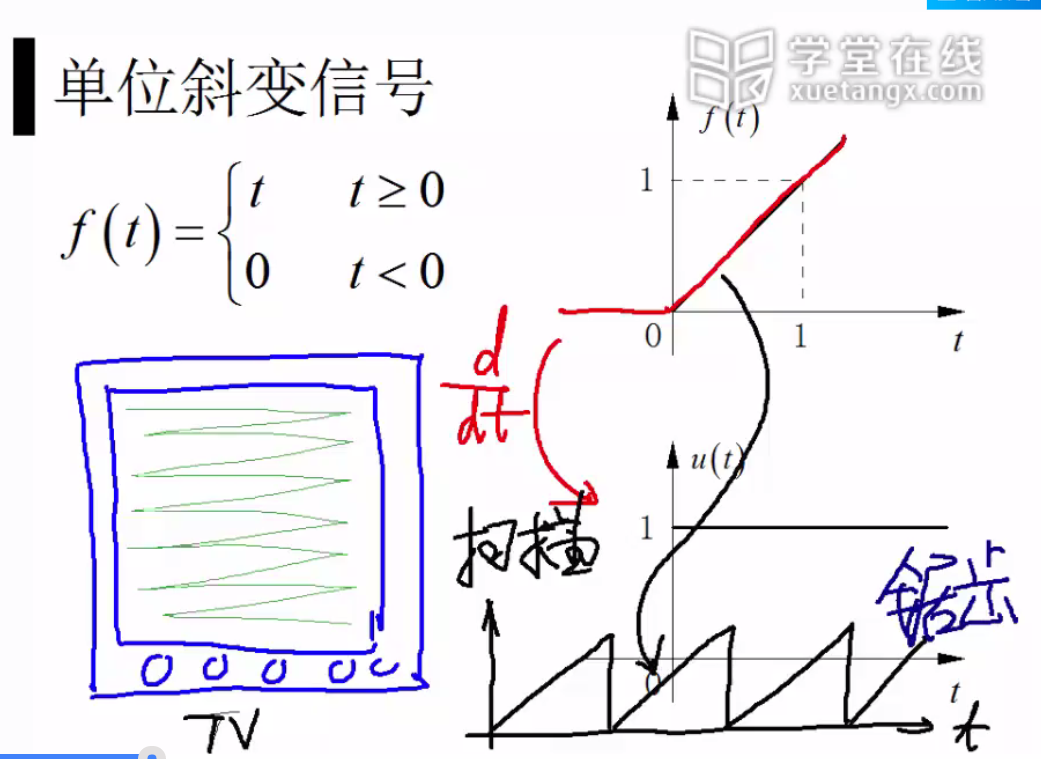### 多项式因果信号

与斜变信号对应的是多项式因果信号，这些多项式信号经过多次求导之后也会变为不连续的信号。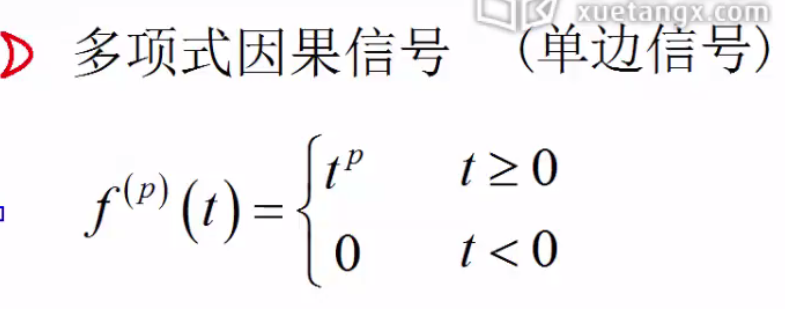### 单位阶跃信号

单位阶跃信号是单位斜变信号求导而得出的结果.我们通常认为在0点处对应的幅值为0.5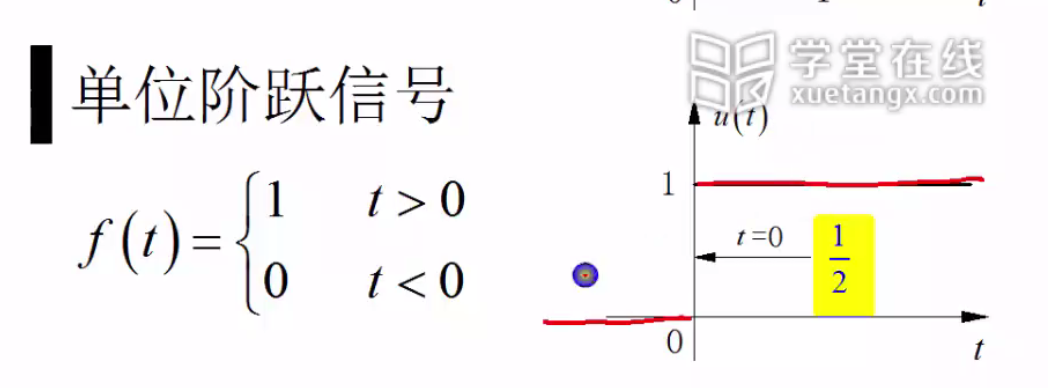### 单位冲击信号

单位阶跃信号再求导就得到了单位冲击信号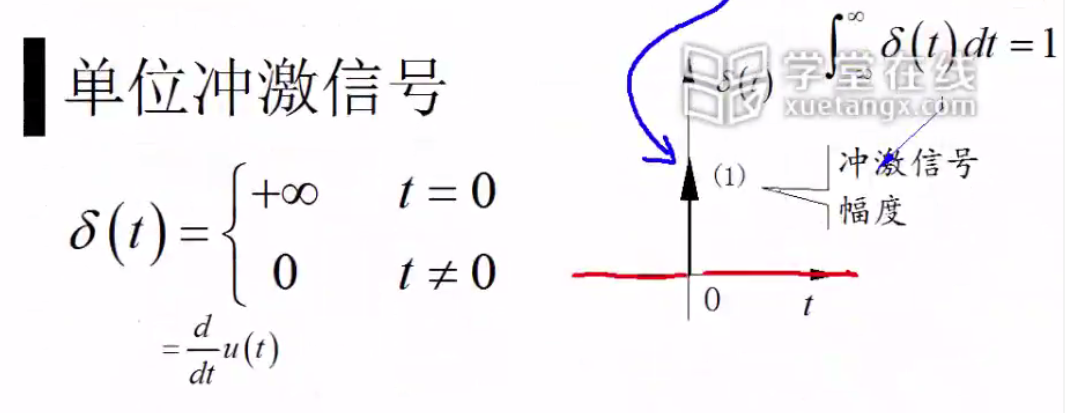### 单位冲击偶信号

我们在单位冲击信号的基础之上再求导，就得到了单位冲击偶信号。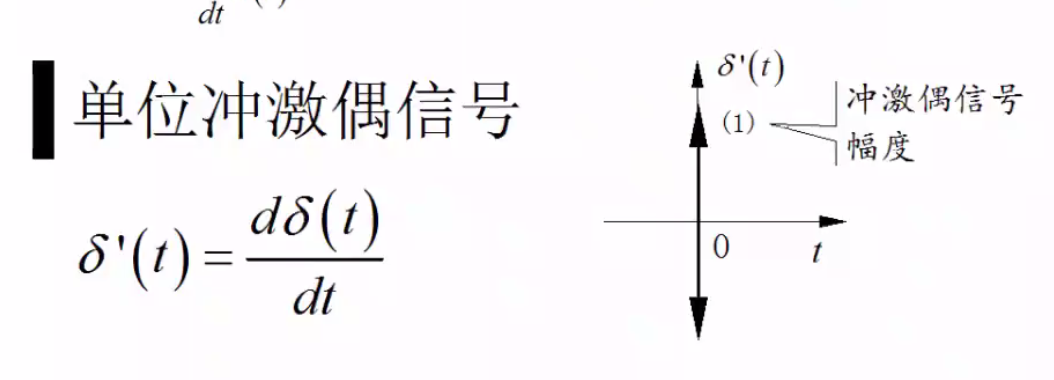最后我们用一张图来概括一下这四类信号，他们之间的关系是逐一求导。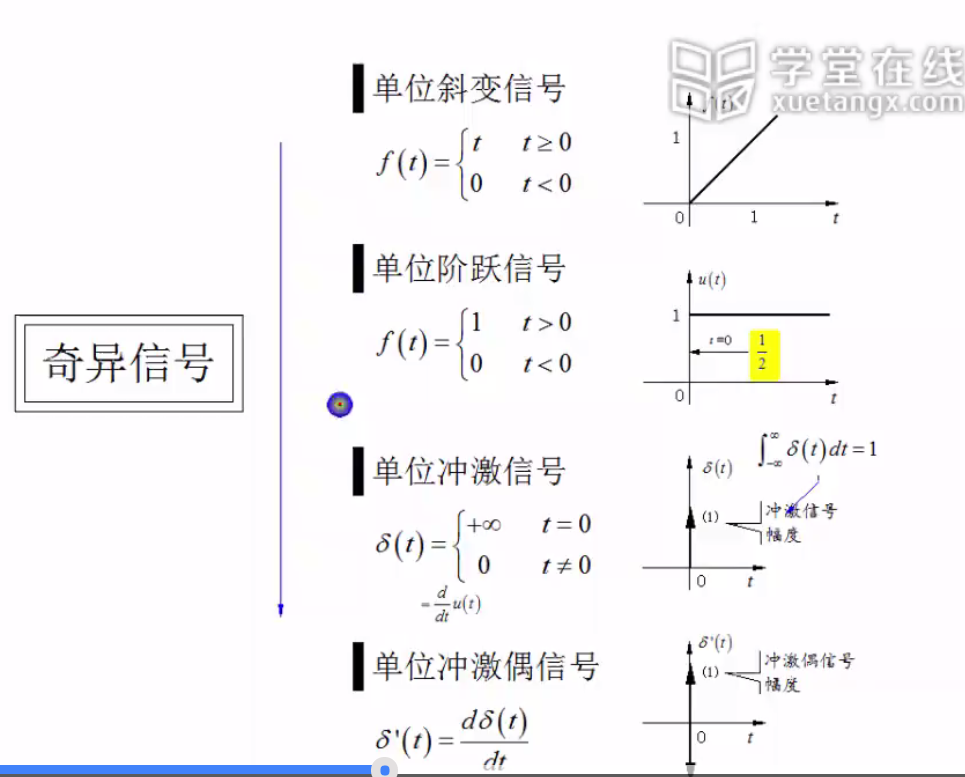### 单位阶跃信号的作用

他与其他信号相乘可以表示信号的起始和结束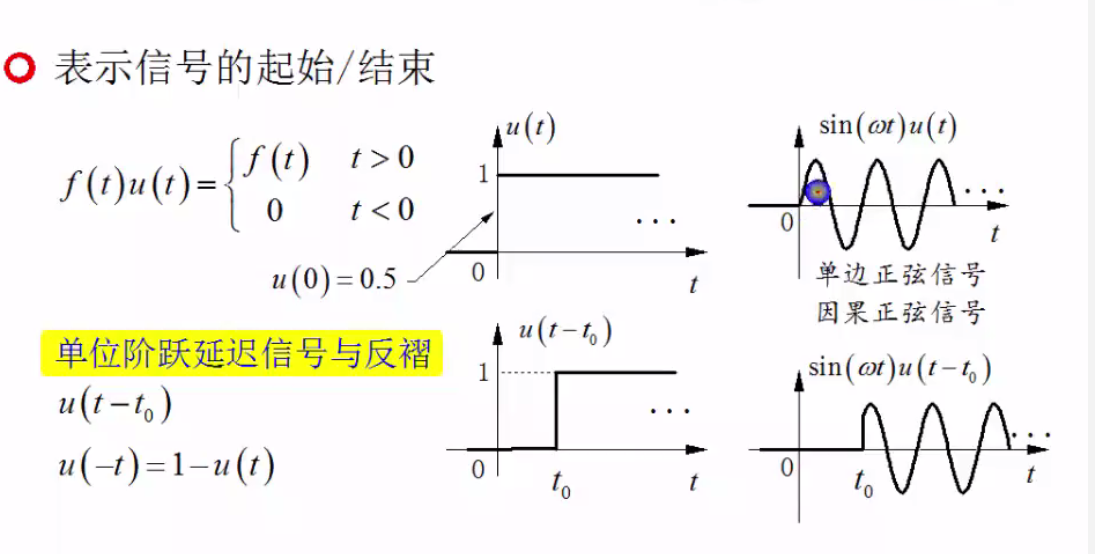也可以和自身相加减之后形成窗口信号，可以用窗口信号来截取别的信号。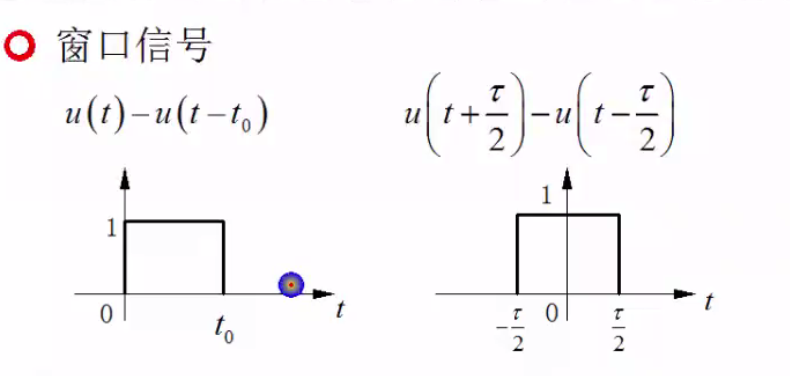下图为截取信号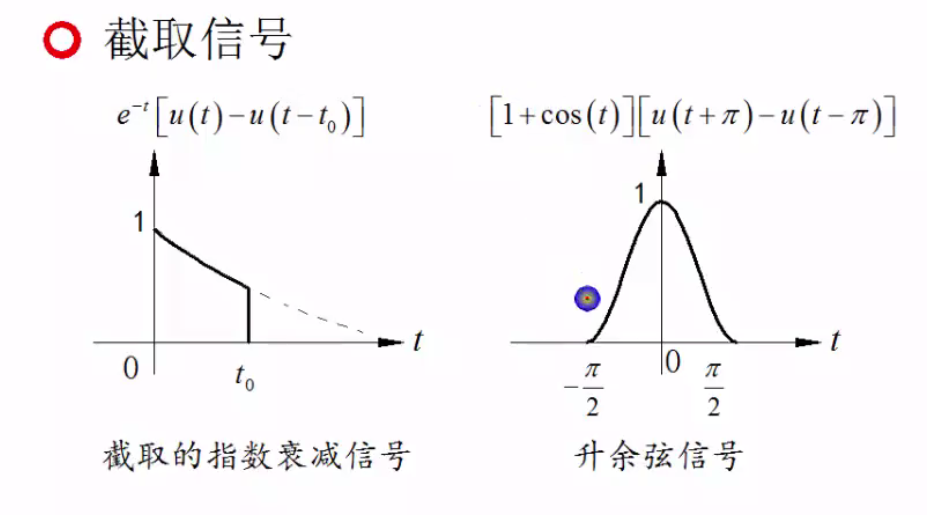在窗口信号的基础之上，我们可以进一步的形成分段信号，分段信号可以写成窗口信号的组合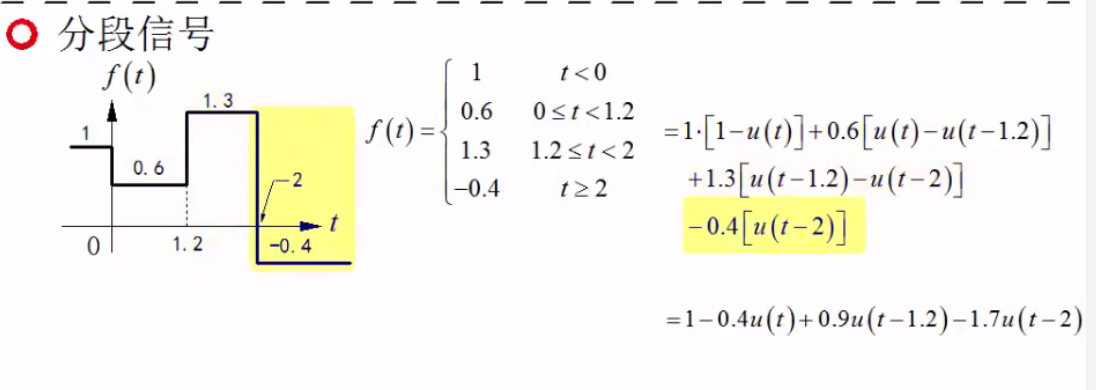单位阶跃信号还和符号函数有着密切的关系，如图所示，二者实际上是满足线性关系的。

## 连续时间奇异信号

虽然单位冲激函数是单位阶跃函数的导数，但是不是很好理解，我们可以这样理解。想象一个面积为1的长方形，当他的底为0的时候高度为无穷大。

因为单位冲激函数和狄克拉函数的定义一样，所以我们有的时候也称单位冲激函数为derda函数。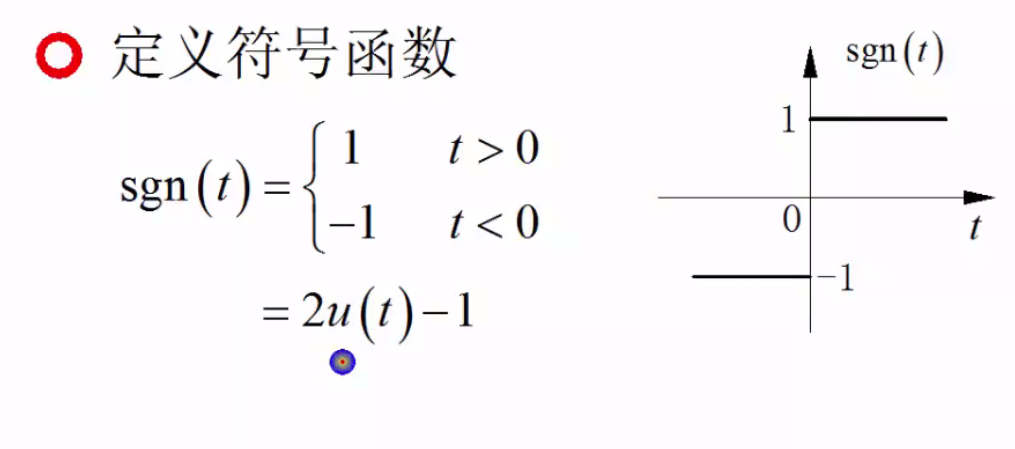### 单位冲击信号的数学特性

如图所示，单位冲击信号与任意信号的积分实际上是任意信号在零点的取值。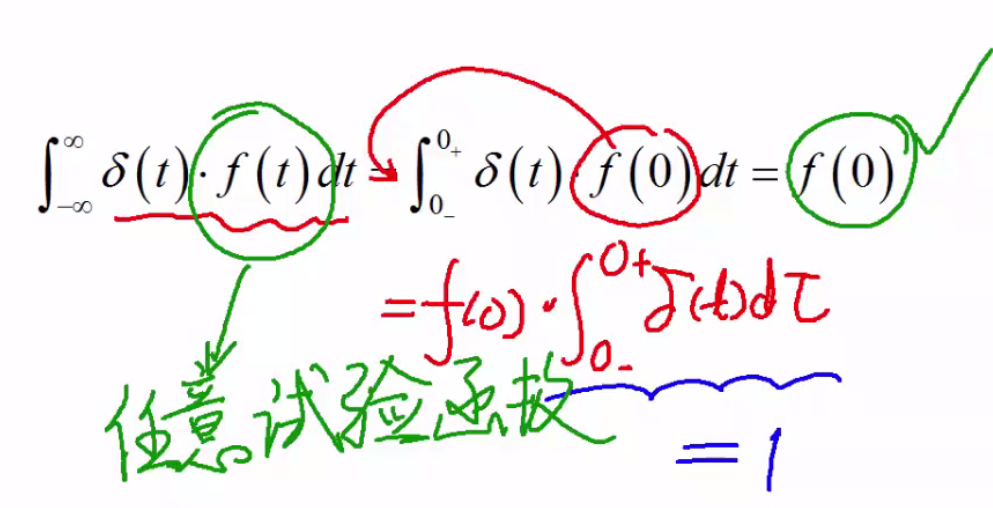我们根据此公式反过来也可以证明冲击信号，也就是满足这个条件的信号就是冲击信号。

他还有这样的性质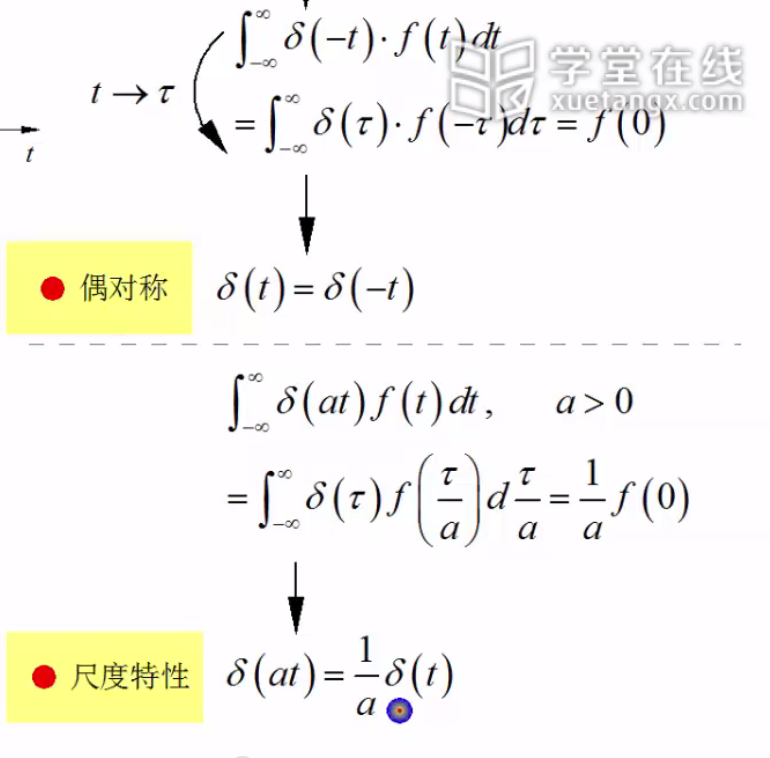如下图所示，可以进行这样的变换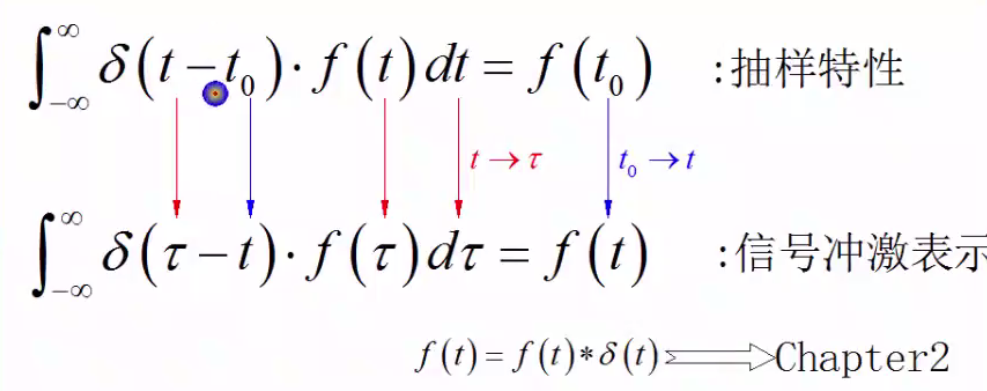最后我们总结一下冲激函数和冲击偶函数的性质：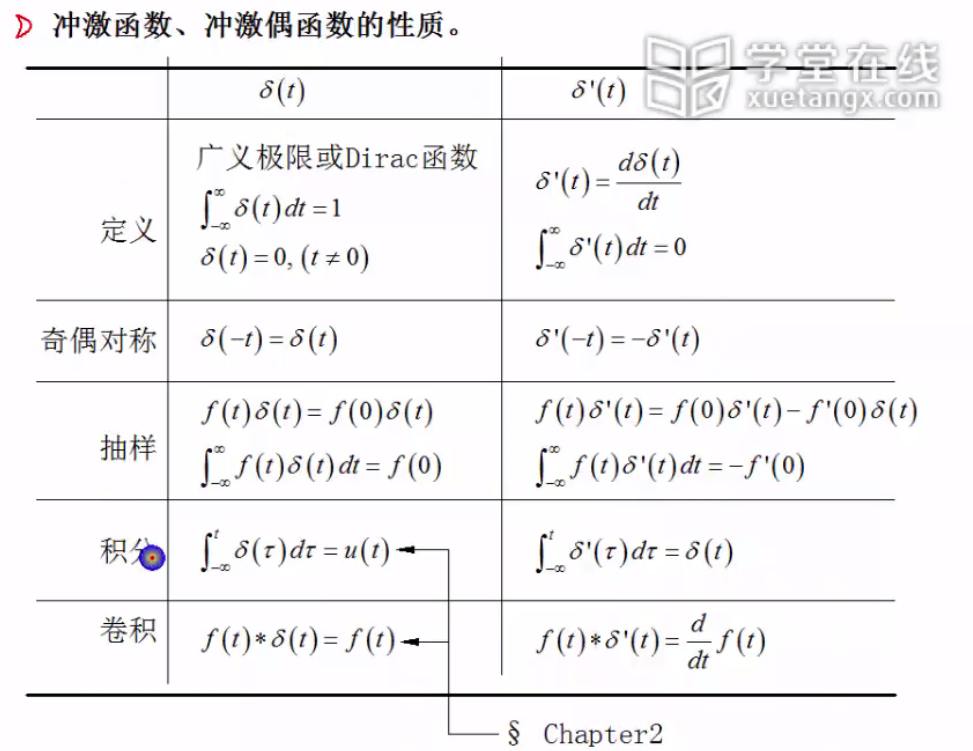## 离散时间奇异信号

对于离散时间信号我们和连续时间信号比较着来学习如图所示，二者之间可以相互转化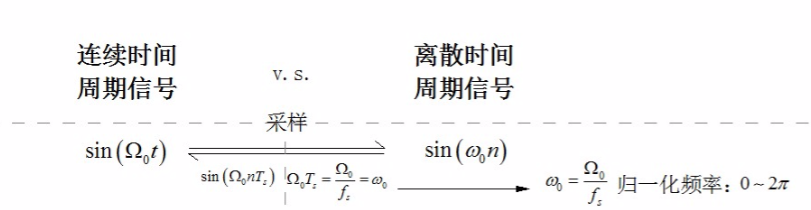如下图所示，对于连续周期信号而言，他总是周期信号。但是对于序列信号不一定。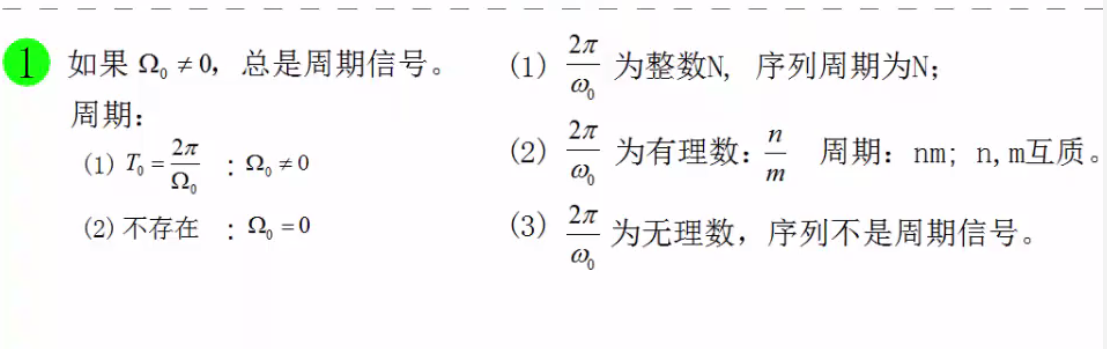序列信号可以额看成是周期的包络线信号和周期的脉冲信号的乘积。两个周期信号的复合信号有可能是一个周期信号，也有可能是一个非周期的信号。对于序列信号来说，只有当他的频率与 π $\pi$的比值为有理数的时候，他才是严格意义上的周期信号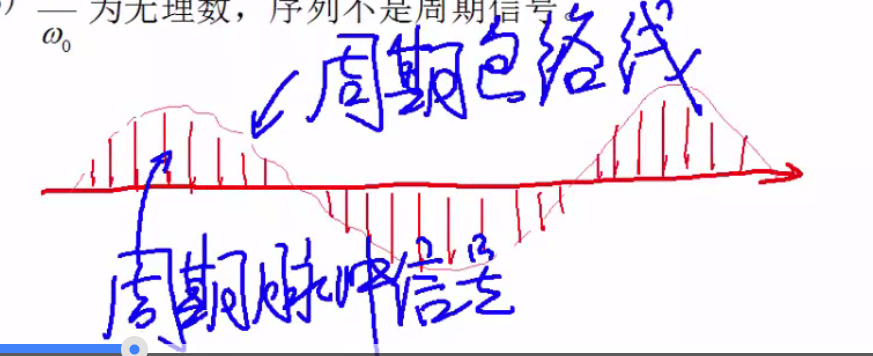对于连续周期信号我们知道随着频率的增加，他的波形变得越来越密。但是对于离散周期信号并不是这样。如图所示，随著频率的增加，它展现了一种周期性。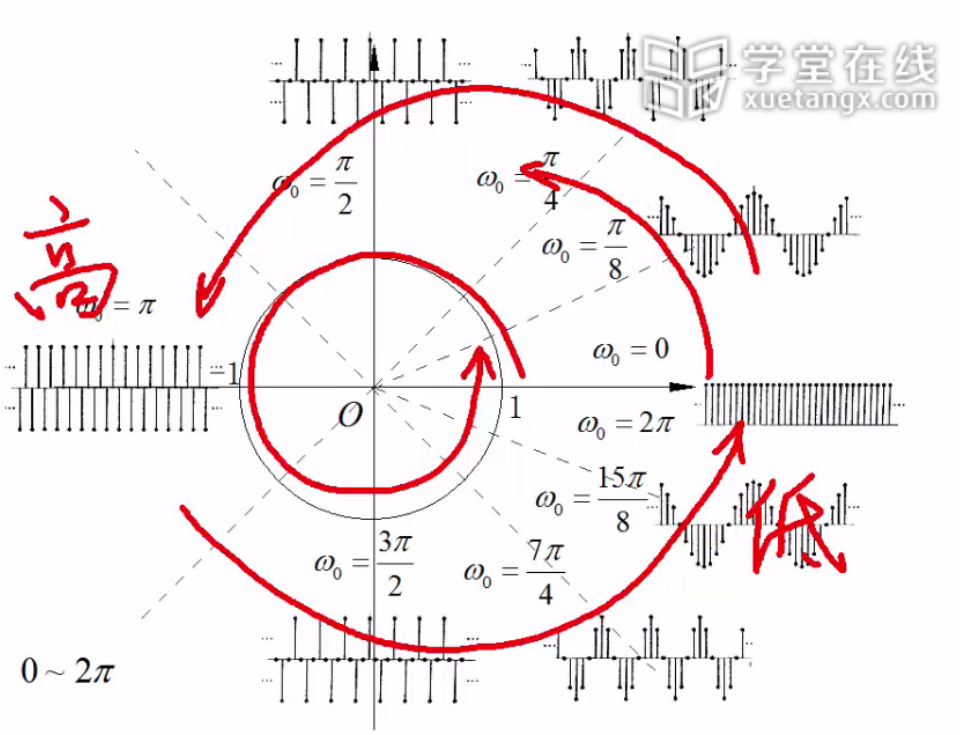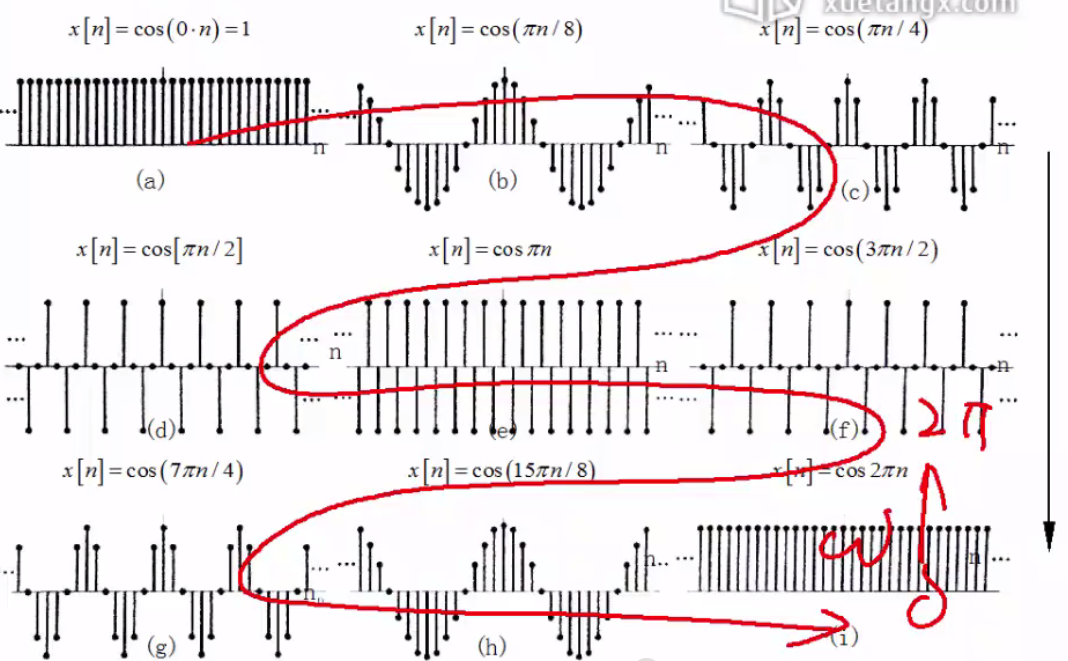另外对于连续的单位冲击信号，在0点的取值是无穷大，但是对于离散的信号序列而言是1.连续的单位阶跃信号在0点的取值是1/2，离散的信号序列在0点取值是1

## 练习题展开全文• 用一个时间函数或一条曲线来表示信号随时间变化的特性称为连续信号的时域描述。在多种多样的连续确定性信号中，有一些信号可以用常见的基本函数表示，如正弦函数、指数函数、阶跃函数等，这类信号为基本信号，可组成...

连续的确定性信号是可用时域上连续的确定性函数描述的信号，是一类在描述、分析上最简单的信号，同时又是其他信号分析的基础。

通常一个信号是时间的函数，在时间域内对其进行定量和定性的描述、分析是一种最基本的方法

### 一、连续信号的时域描述

用一个时间函数或一条曲线来表示信号随时间变化的特性称为连续信号的时域描述。在多种多样的连续确定性信号中，有一些信号可以用常见的基本函数表示，如正弦函数、指数函数、阶跃函数等，这类信号为基本信号，可组成复杂信号，分为普通信号奇异信号

（一）普通信号的时域描述

1.正弦信号

• 余弦信号也是正弦信号

• 如果一个正弦信号的频率是 f 1 f_1 是另一个正弦信号频率 f 0 f_0 的整数倍，即 f 1 = n f 0 f_1=nf_0 （n为整数），则其合成信号是频率为 f 0 f_0 的非正弦周期信号，把 f 0 f_0 称为该信号的基波频率， f 1 f_1 称为n次谐波频率。据此，可以把一个周期信号分解为基波信号和一系列谐波信号。

2.指数信号

表示为
x ( t ) = A e s t − ∞ < t < ∞ x(t)=Ae^{st} \quad -\infty<t<\infty
式中， s = σ + j ω s=\sigma+j\omega 为复数。

如果 σ = 0 , ω = 0 \sigma=0,\omega=0 ，则 x ( t ) = A x(t)=A 即为直流信号。

如果 σ ≠ 0 , ω = 0 \sigma \neq 0, \omega=0 ，则 x ( t ) = A e σ t x(t)=Ae^{\sigma t} ，即为实指数信号，其中信号的衰减或增长速度可以用实指数信号的时间常数 τ \tau 表示，它是 ∣ σ ∣ |\sigma| 的倒数，即 τ = 1 ∣ σ ∣ \tau=\frac{1}{|\sigma|}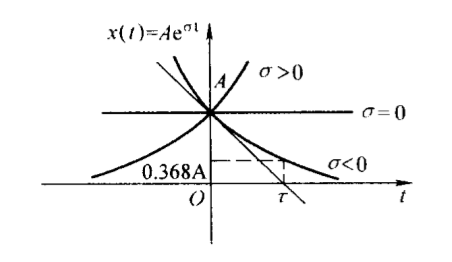如果 σ ≠ 0 , ω ≠ 0 \sigma \neq 0,\omega \neq 0 ，则 x ( t ) = A e σ t e j ω t x(t)=Ae^{\sigma t}e^{j\omega t} ，即为复指数信号， s = σ + j ω s=\sigma+j\omega 称为复指数信号的复频率。

按欧拉（Euler）公式，复指数信号可以写成
x ( t ) = A e s t = A e σ t e j ω t = A e σ t c o s ω t + j A e a t s i n ω t = R e [ x ( t ) ] + j I m [ x ( t ) ] x(t)=Ae^{st}=Ae^{\sigma t}e^{j\omega t}=Ae^{\sigma t}cos\omega t+jAe^{at}sin\omega t=Re[x(t)]+jIm[x(t)]
x ( t ) x(t) 可以分解为实部和虚部两个部分
R e [ x ( t ) ] = A e σ t c o s w t I m [ x ( t ) ] = A e σ t s i n w t Re[x(t)]=Ae^{\sigma t}coswt \\ Im[x(t)]=Ae^{\sigma t}sinwt
分别为余弦和正弦信号， A e σ t Ae^{\sigma t} 反映了它们振荡幅度的变化情况，即它们的包络线。下图表示了 σ < 0 \sigma<0 时的 R e [ x ( t ) ] Re[x(t)] I m [ x ( t ) ] Im[x(t)] ，其中虚线为包络线 A e σ t Ae^{\sigma t}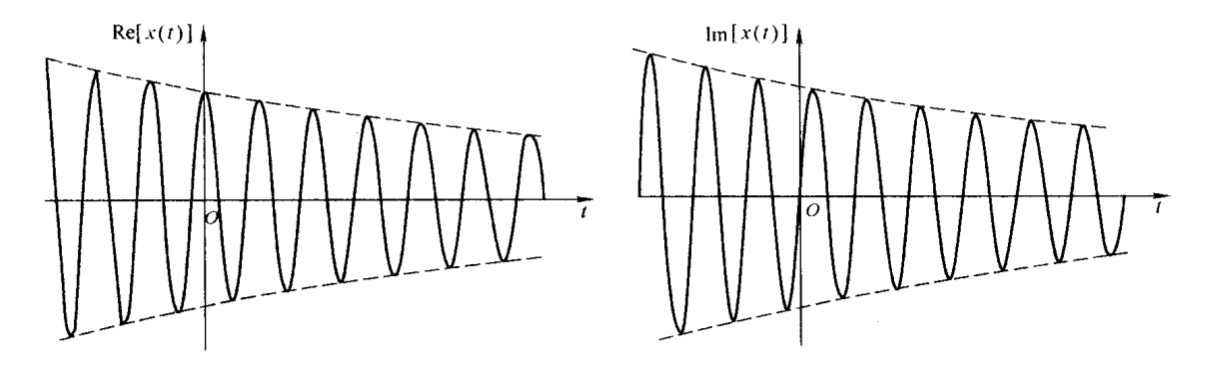实际的信号总是实的，即都是时间t的实函数，复指数信号为复函数，所以不可能实际产生。但是一方面，它的实部和虚部表示了指数包络的正弦型振荡，具有一定的实际意义。其次，它把直流信号、指数信号、正弦型信号以及具有包络线的正弦型信号表示为统一的形式，在信号分析理论中更具有普遍意义。

欧拉公式：
e j w t = c o s w t + j s i n w t A c o s ( w t + φ ) = A 2 [ e j ( w t + φ ) + e − j ( w t + φ ) ] = A R e [ e j ( w t + φ ) ] A s i n ( w t + φ ) = A 2 j [ e j ( w t + φ ) − e − j ( w t + φ ) ] = A I m [ e j ( w t + φ ) ] e^{jwt}=coswt+jsinwt \\ Acos(wt+\varphi)=\frac{A}{2}[e^{j(wt+\varphi)}+e^{-j(wt+\varphi)}]=ARe[e^{j(wt+\varphi)}] \\ Asin(wt+\varphi)=\frac{A}{2j}[e^{j(wt+\varphi)}-e^{-j(wt+\varphi)}]=AIm[e^{j(wt+\varphi)}]
（二）奇异信号的描述

奇异信号是用奇异函数表示的一类特殊的连续时间信号，其函数本身或者函数的导数（包括高阶导数）具有不连续点。它们是从实际信号中抽象出来的典型信号，在信号的分析中占有重要地位。

1. 单位斜坡信号
2. 单位阶跃信号

阶跃信号具有单边特性，即信号在接入时刻 t 0 t_0 以前的值为0，因此，可以用来描述信号的接入特性，如 x ( t ) = s i n w t ⋅ u ( t ) x(t)=sinwt·u(t)

通过阶跃信号，可以表示出矩形脉冲信号。
x ( t ) = A [ u ( t + τ 2 ) − u ( t − τ 2 ) ] x(t)=A[u(t+\frac{\tau}{2})-u(t-\frac{\tau}{2})]

1. 单位冲击信号

狄拉克把单位冲激信号定义为
{ δ ( t ) = 0 , t ≠ 0 ∫ − ∞ ∞ δ ( t ) d t = 1 (1) \begin{cases} \delta(t)=0, \quad t\neq 0 \\ \int_{-\infty}^{\infty}\delta(t)dt=1 \end{cases} \tag{1}
即非零时刻的函数值均为零，而它与时间轴覆盖的面积为1。为了方便理解，可以把单位冲激信号视为幅度为 1 τ \frac{1}{\tau} 、宽度为 τ \tau 的矩形脉冲当 τ \tau 趋于零时的极限情况，即
δ ( t ) = l i m τ → 0 1 τ [ u ( t + τ 2 ) − u ( t − τ 2 ) ] \delta(t)=lim_{\tau\to 0}\frac{1}{\tau}[u(t+\frac{\tau}{2})-u(t-\frac{\tau}{2})]
下图表示了 τ → 0 \tau \to 0 时上述矩形脉冲的变化过程。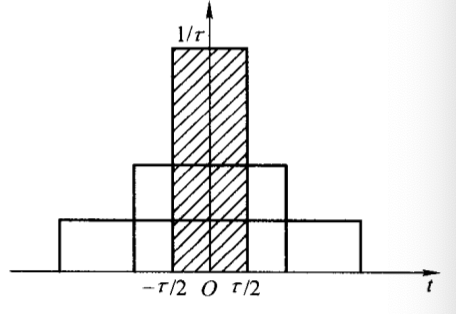由上可知，当 t = 0 t=0 时， δ ( t ) \delta(t) 的幅值应为 ∞ \infty ，无明确的物理意义。但是由式（1）， ∫ − ∞ ∞ δ ( t ) d t = ∫ 0 − 0 + δ ( t ) d t = 1 \int_{-\infty}^{\infty}\delta(t)dt=\int_{0^-}^{0^+}\delta(t)dt=1 ，故称 δ ( t ) \delta(t) 的强度为1，用带箭头的直线段表示，并在箭头旁边标以强度1.如下图所示。如果一个冲激信号与时间轴覆盖的面积为A，表示其强度是单位冲激信号的A倍，用在带箭头的直线段旁边标以A来表示。

冲激信号的性质：

1）若 x ( t ) x(t) t = 0 t=0 处连续，则有
∫ − ∞ ∞ x ( t ) δ ( t ) d t = x ( 0 ) (2) \int_{-\infty}^{\infty}x(t)\delta(t)dt=x(0) \tag{2}
这是因为 δ ( t ) \delta(t) t ≠ 0 t\neq 0 处为零，故有
∫ − ∞ ∞ x ( t ) δ ( t ) d t = ∫ 0 − 0 + x ( t ) δ ( t ) d t = x ( 0 ) ∫ 0 − 0 + δ ( t ) d t = x ( 0 ) \int_{-\infty}^{\infty}x(t)\delta(t)dt=\int_{0^-}^{0^+}x(t)\delta(t)dt=x(0)\int_{0^-}^{0^+}\delta(t)dt=x(0)
一个任意信号 x ( t ) x(t) 经与 δ ( t ) \delta(t) 相乘后再取积分，就是该信号在 t = 0 t=0 处的取值，表明 δ ( t ) \delta(t) 具有取样（筛选）特性。

2）冲激信号具有偶函数特性，这是因为如令 τ = − t \tau=-t ，则有
∫ − ∞ ∞ x ( t ) δ ( − t ) d t = ∫ − ∞ ∞ x ( − τ ) δ ( τ ) d ( − τ ) = ∫ ∞ − ∞ x ( − τ ) δ ( τ ) d τ = x ( 0 ) \int_{-\infty}^{\infty}x(t)\delta(-t)dt=\int^\infty_{-\infty}x(-\tau)\delta(\tau)d(-\tau)=\int_{\infty}^{-\infty}x(-\tau)\delta(\tau)d\tau=x(0)
再结合式（2），有
δ ( − t ) = δ ( t ) \delta(-t)=\delta(t)
3）冲激信号与阶跃信号互为积分和微分关系，即
∫ − ∞ t δ ( τ ) d τ = u ( t ) ( 3 ) d u ( t ) d t = δ ( t ) ( 4 ) \int_{-\infty}^t\delta(\tau)d\tau=u(t) \quad (3)\\ \frac{du(t)}{dt}=\delta(t) \quad (4)
这是因为由冲激信号的定义式（1）有
∫ − ∞ t δ ( τ ) d τ = { ∫ − ∞ ∞ δ ( τ ) d τ = 1 t > 0 0 t < 0 \int_{-\infty}^t\delta(\tau)d\tau= \begin{cases} \int_{-\infty}^{\infty}\delta(\tau)d\tau=1 \quad t>0 \\ 0 \quad t<0 \end{cases}
结合 u ( t ) u(t) 的定义式，即可得式（3）、（4）。

### 二、连续信号的时域运算

尺度变换、平移、翻转、叠加、相乘、微分、积分等

1. 尺度变换

幅度变换不改变信号的基本特性，如果 x ( t ) x(t) 表示某一语音信号，则 x 1 ( t ) x_1(t) x 2 ( t ) x_2(t) 仅仅使声音的大小发生了变化，语音特征并没有变化。时间尺寸会改变信号的基本特征，信号的频谱发生了变化。声音音调的变化是由于信号的频率特性发生变化。信号的频率特性与幅度不同，它是信号的基本特征。

2. 微分和积分

单位冲激信号的微分
δ ′ ( t ) = d d t δ ( t ) \delta'(t)=\frac{d}{dt}\delta(t)
可视为幅度为 1 τ \frac{1}{\tau} ，脉宽为 τ \tau 的矩形脉冲求导后 τ \tau 趋于零的极限。显然，它是位于 t = 0 t=0 处强度分别为 + ∞ +\infty − ∞ -\infty 的一对冲激函数，故称为单位冲激偶，如下图所示。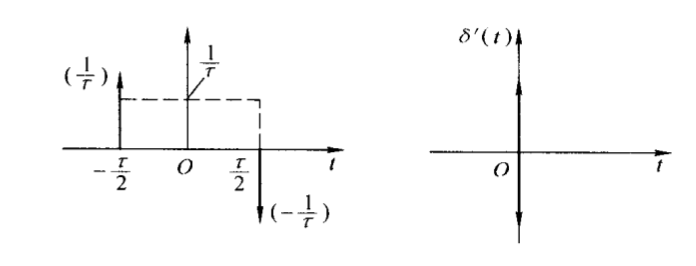单位冲激偶函数是奇函数，即
∫ − ∞ + ∞ δ ′ ( t ) = 0 \int_{-\infty}^{+\infty}\delta'(t)=0
这可由 δ ′ ( t ) \delta'(t) 的定义直接得到。此外单位冲激偶函数也有筛选特性
∫ − ∞ + ∞ x ( t ) δ ′ ( t − t 0 ) d t = − x ′ ( t 0 ) \int_{-\infty}^{+\infty}x(t)\delta'(t-t_0)dt=-x'(t_0)

1. 卷积运算

对于两个连续时间信号，卷积运算为
x 1 ( t ) ∗ x 2 ( t ) = ∫ − ∞ ∞ x 1 ( τ ) x 2 ( t − τ ) d τ = ∫ − ∞ ∞ x 2 ( τ ) x 1 ( t − τ ) d τ x_1(t)*x_2(t)=\int_{-\infty}^{\infty}x_1(\tau)x_2(t-\tau)d\tau=\int_{-\infty}^{\infty}x_2(\tau)x_1(t-\tau)d\tau
显然 x 1 ( t ) ∗ x 2 ( t ) = x 2 ( t ) ∗ x 1 ( t ) x_1(t)*x_2(t)=x_2(t)*x_1(t)

任意信号与冲激信号的卷积有特殊意义。首先，任意 x ( t ) x(t) 与单位冲激信号 δ ( t ) \delta(t) 的卷积仍然是 x ( t ) x(t) 本身，这是因为
x ( t ) ∗ δ ( t ) = ∫ − ∞ ∞ x ( τ ) δ ( t − τ ) d τ = ∫ − ∞ ∞ x ( τ ) δ ( τ − t ) d τ = x ( t ) x(t)*\delta(t)=\int_{-\infty}^{\infty}x(\tau)\delta(t-\tau)d\tau=\int_{-\infty}^{\infty}x(\tau)\delta(\tau-t)d\tau=x(t)
其次，有
x ( t ) ∗ δ ( t − t 0 ) = ∫ − ∞ ∞ x ( τ ) δ ( t − t 0 − τ ) d τ = ∫ − ∞ + ∞ x ( τ ) δ ( τ − ( t − t 0 ) ) d τ = x ( t − t 0 ) x(t)*\delta(t-t_0)=\int_{-\infty}^{\infty}x(\tau)\delta(t-t_0-\tau)d\tau=\int_{-\infty}^{+\infty}x(\tau)\delta(\tau-(t-t_0))d\tau=x(t-t_0)
δ ( t − t 0 ) \delta(t-t_0) 卷积，相当于原信号延迟 t 0 t_0 ，进一步，有
x ( t − t 1 ) ∗ δ ( t − t 2 ) = ∫ − ∞ + ∞ x ( τ − t 1 ) δ ( t − t 2 − τ ) d τ = ∫ − ∞ + ∞ x ( τ − t 1 ) δ ( τ − ( t − t 2 ) ) d τ = ∫ − ∞ + ∞ x ( λ ) δ ( τ − ( t − t 1 − t 2 ) ) d λ = x ( t − t 1 − t 2 ) x(t-t_1)*\delta(t-t_2)=\int_{-\infty}^{+\infty}x(\tau-t_1)\delta(t-t_2-\tau)d\tau \\ =\int_{-\infty}^{+\infty}x(\tau-t_1)\delta(\tau-(t-t_2))d\tau \\ =\int_{-\infty}^{+\infty}x(\lambda)\delta(\tau-(t-t_1-t_2))d\lambda \\ =x(t-t_1-t_2)

展开全文• 文章目录一、四类典型信号1.1 连续时间和离散时间的指数信号1.2 连续时间和离散时间的正弦信号1.3 连续时间和离散时间的单位冲激信号和单位阶跃信号1.3.1 单位冲激函数的作用：1.4 Sa信号（sinc信号） 一、四类典型...
• 2． 熟悉连续信号经过采样前后的频谱变化，加深对采样定理的理解。3． 掌握 MATLAB 产生常用离散时间信号的编程方法。二、实验原理1． 单位抽样序列: 01)(n在 MATLAB 中可以利用 zeros()函数实现。 ;1)...
• 2.1.4 离散信号的描述 无论是采样得到的离散信号，还是客观事物给出的离散信号，只要给出函数值的离散时刻是等间隔的，我们都可以用序列x(n)x(n)x(n)来表示它们，这里n是各函数值在序列中出现的序号。 通常可以用x(n...
• 连续非周期信号频谱分析及Matlab 实现 连续非周期信号频谱分析及 MATLAB 实现 谢海霞 1，孙志雄 1 (琼州学院海南 三亚 572022) 摘要：为了便于计算机辅助计算复杂的连续信号频谱，常常采用DFT方法。DFT不仅能反映...
• 在分析典型机械故障声发射信号特征的基础上，根据损伤型声发射信号故障特征的提取原理，通过连续小波基函数的构造方法，设计了一种适于声发射信号故障特征提取的小波基函数。将该函数与普遍使用的Daubechies小波同时...
• 语音信号处理主要包括语音通信(语音编码)，语音合成和语音识别等，前提是对语音信号进行分析。只有将语音信号分析表示成其本质特性的参数，才有可能利用这些参数进行高效的语音通信，才可能建立用于语音合成的语音库...语音识别
• 幅值连续:不同时间点之间的幅度值之...连续时间信号: 时间连续，幅值可以连续也可以离散； 离散时间信号：时间离散，幅值可以离散也可以连续； 模拟信号: 时间连续，幅值连续； 数字信号; 时间离散，幅值离散： ...
• 深入研究连续时间信号傅里叶级数分析的理论知识，利用MATLAB强大的图形处理功能，符号运算功能以及数值计算功能，实现连续时间周期信号频域分析的仿真波形。 1.用MATLAB实现周期信号的傅里叶级数分解与综合。 2.用...
• 连续时间信号时间连续幅值连续或离散 模拟信号时间连续幅值连续上种信号的特例 离散时间信号序列时间离散幅值连续 数字信号时间离散幅值量化 系统 按处理的信号种类不同可将系统分为 连续时间系统 模拟系统 离散时间...
• 连续时间和离散时间信号 一个信号，它是在时间t的连续值上给出的，...术语连续时间和离散时间是根据信号沿时间（水平）轴的特征来认定的；而术语模拟和数字则是依据信号的幅度（垂直轴）属性判定的。模拟的不一定是连
• 实验一常用连续时间信号的实现 实验目的: 熟悉 MATLAB的基本使用方法 典型信号的 MATLAB表示方法 掌握 MATLABI函数的应用 熟悉 MATLAB的基本使用方法 MATLAB是矩阵实验室( Matrix Laboratory)之意 除具备卓越的数值...
• 课程设计任务书 学生 专业班级电子科学与技术0803 班 指导教师 工作单位 信息工程学院 题 目: 连续时间信号的傅利叶变换及MATLAB实现 初始条件 MATLAB软件微机 要求完成的主要任务: 利用MATLAB强大的图形处理功能...
• 学 海 无 涯 非周期信号的傅里叶变换MATLAB 仿真实验 一实验目的 1熟悉连续非周期信号频谱特点及其分析方法 2 掌握用MATLAB 实现傅里叶变换 二非周期信号的傅里叶变换原理及性质 设周期信号 f (t) 展开成复指数形式...
• 最后对单载频信号、线性调频信号和非线性调频信号等三种典型连续调频信号进行了仿真分析.仿真结果表明,该方法在保持瞬时自相关方法性能的基础上实现了对非线性调频信号的脉内分析,具有一定的工程应用价值.
• 原标题：典型雷达信号之“相位编码信号”线性调频信号、非线性调频信号调制函数是连续的，属于“连续型”信号，而相位编码信号，其相位调制函数是离散的有限状态，属于“离散型”编码脉冲压缩信号。由于相位编码采用...
• 本次课程重点从模糊函数出发，仿真分析多种典型雷达信号：线性调频脉冲信号、三角波调频连续信号、二相编码信号(Barker码/m序列)、多相编码信号(Frank码)。课程将给出上述典型雷达信号的产生以及模糊图的Matlab...
• （3）熟悉连续信号卷积运算函数conv的应用。 （4）熟悉连续LTI系统在典型激励信号下的响应及其特征； （5）掌握连续LTI系统单位冲激响应的求解方法； （6）掌握用卷积法计算连续时间系统的零状态响应； （7）能够...
• 上机实验 2 连续 LTI系统的时域分析 一实验目的 熟悉 LTI 系统在典型激励信号的响应及其特性 熟悉连续 LTI 系统单位冲激响应的求解方法 重点掌握用卷积计算连续时间系统的零状态响应 熟悉 MATLAB相关函数的调用格式...
• 信号与系统》吴大正公开课随课笔记第二章——讲述了连续时域系统的响应结构及其求解方法。卷积
• 如果T0T_0T0​趋于无穷大时，则周期矩形脉冲信号将演变成非周期的矩形脉冲信号，可以预料，此时谱线会无限密集而演变成为连续的频谱，但与此同时，谱线的幅度将趋于零而变成无穷小量。为了避免在一系列无穷小量中...
• 模拟信号是指用连续变化的物理量表示的信息，其信号的幅度，或频率，或相位均随时间作连续变化，如广播电视中的声音信号，或图像信号等。处理模拟信号的电子电路称为模拟电路。模拟电路主要包括放大电路、振荡电路和...
• 相比于前两篇 BlogBlogBlog 中关于卷积物理意义以及性质的讨论，这篇 BlogBlogBlog 重点归纳卷积计算的技巧和方法。...在着手开始分析第一个例子之前，我们回顾一下连续信号的卷积公式：y(t)=∫−∞+∞x(τ)...卷积
• 典型信号傅里叶级数分解周期方波信号如图所示，对于周期方波信号，当他的占空比为半分之五十的时候，他的信号形式是这样的，此时如果n为偶数的时候，他的an为0。也就是只有奇数次项才有波形。我们称此为奇谐波我们...
• 针对这个问题，分析信号的特点，对几种典型的时频分析法进行比较，提出了用连续小波变换时频法分析射手失误时的信号。通过Matlab仿真实验证明，笔者提出的方法对射手失误信号的处理是有效的，能准确地确定失误的位置......